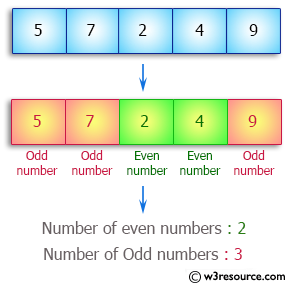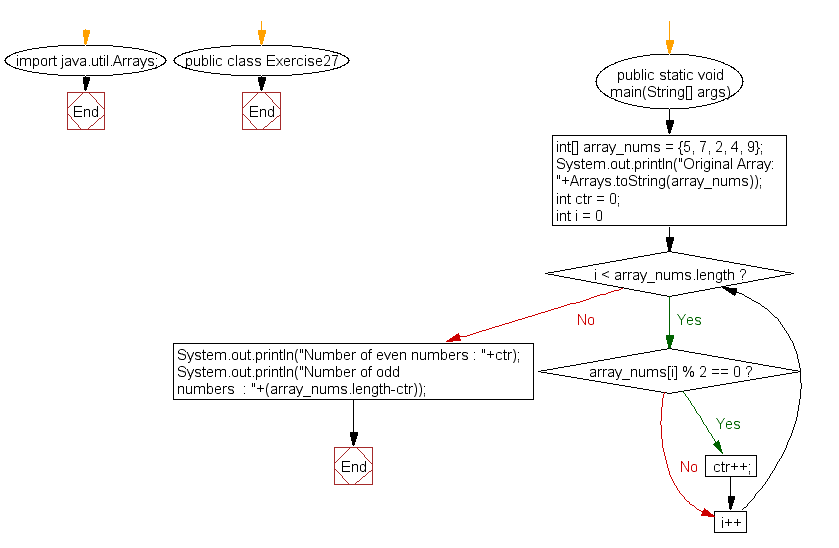﻿ Java: Find the number of even and odd integers in a array# Java Array Exercises: Find the number of even and odd integers in a given array of integers

## Java Array: Exercise-27 with Solution

Write a Java program to find the number of even and odd integers in a given array of integers.

Pictorial Presentation:Sample Solution:

Java Code:

``````import java.util.Arrays;
public class Exercise27 {
public static void main(String[] args)
{
int[] array_nums = {5, 7, 2, 4, 9};
System.out.println("Original Array: "+Arrays.toString(array_nums));
int ctr = 0;
for(int i = 0; i < array_nums.length; i++)
{
if(array_nums[i] % 2 == 0)
ctr++;
}
System.out.println("Number of even numbers : "+ctr);
System.out.println("Number of odd numbers  : "+(array_nums.length-ctr));
}
}
```
```

Sample Output:

```
Original Array: [5, 7, 2, 4, 9]
Number of even numbers : 2
Number of odd numbers  : 3
```

Flowchart:Visualize Java code execution (Python Tutor):

Java Code Editor:

Improve this sample solution and post your code through Disqus

What is the difficulty level of this exercise?

Test your Programming skills with w3resource's quiz.

﻿

## Java: Tips of the Day

System.currentTimeMillis vs System.nanoTime

In Java, there are two standard ways of conducting time-operations and it is not always clear which one should be chosen.

The method System.currentTimeMillis() returns the current number of milliseconds since the beginning of the Unix era in the format Long. Its accuracy ranges from 1 to 15 thousandths of a second, depending on the system.

`long startTime = System.currentTimeMillis();long estimatedTime = System.currentTimeMillis() - startTime;`

The method System.nanoTime has an accuracy of up to one-millionth of a second (nanoseconds) and returns the current value of the most accurate available system timer.

```long startTime = System.nanoTime();long estimatedTime = System.nanoTime() - startTime;
```

Thus, it is System.currentTimeMillisexcellent for displaying and synchronizing absolute time, and System.nanoTime for measuring relative intervals.

Ref: https://bit.ly/2W4V97w